Courses

# Raoult's Law Class 12 Notes | EduRev

## Chemistry Class 12

Created by: Mohit Rajpoot

## Class 12 : Raoult's Law Class 12 Notes | EduRev

The document Raoult's Law Class 12 Notes | EduRev is a part of the Class 12 Course Chemistry Class 12.
All you need of Class 12 at this link: Class 12

RAOULT’S LAW

• Let two volatile liquids A and B dissolve each other to form an ideal solution. Then the vapours above the solution will contain the vapours of A and B.
• Applying Dalton’s law of partial pressure the total vapour pressure of the solution will be Psolution = PA + PB
• Where PA and PB are the partial vapour pressures of A and B.
• Solution being dilute (Condition for ideality) Raoult’s law can be applied.
• Raoult’s law for binary solutions state that “the vapour pressure of any volatile constituent of a binary solution at any given temperature is equal to the product of the vapour pressure of pure constituent and its mole fraction”.
PA = PºA XA
PB = PºB XB
• PºA and PºB are vapour pressures of pure A and B  respectively
• xA and xB are mole fraction of A and B in liquid solution respectively
• Hence
• 𝑃 (Solution) 𝑜𝑟 Ps =   PA0 XA + PB0 XB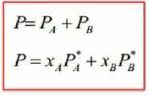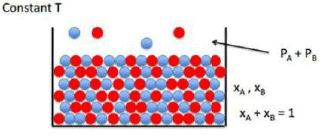• Also,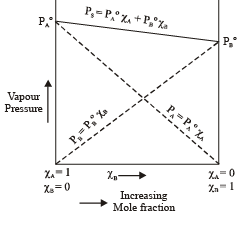• Ps = P0A(1-XB) + P0BXB
•  Ps = P0A+(P0B - P0A)XB
• This equation is of the form y = mx + c
• The value of m (slope) may be (+ve) or (–ve) depending upon whether P0B > P0A (m = + ve) or
• P0B < P0A (m = –ve).
• Hence a plot of a graph of Ps versus XB will be a straight line with an intercept on y-axis equal to pA and slope equal to (P0B - P0A)

Total Pressure of a Solution will be:

• P (Solution) = PA + PB = xAP0+ xBP0B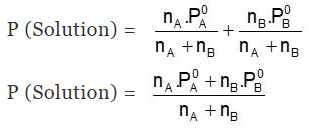VAPOUR COMPOSITION

• Vapour composition means to find out the mole fraction of A and B in vapour i.e yA and yB
• Dalton’s law of particle pressure is used to calculate the vapour composition, as we know
• Partial pressure = mole fraction × total pressure
• pA= yA × pS
• or y! =𝑃A/𝑃𝑆
• Similarly for B
• yB = 𝑃A/𝑃𝑆
• where yA and yB are mole fraction of ‘A’ and ‘B’ in vapour phase and yA +  yB = 1

SPECIAL NOTE

• xA and xB are mole fraction of ‘A’ and ‘B’ in solution phase and
o xA +  xB =1
• yA and yB are mole fraction of ‘A’ and ‘B’ in vapour phase and
o yA +  yB =1

Raoult's Law as a special case of Henry's law

• At a given temperature liquids vaporize. At equilibrium the pressure exerted by the vapour of the liquid over the liquid phase is referred to as vapour pressure.
• According to Raoult’s law, vapour pressure of a volatile component in a given solution ca be defined by pi = pi˚xi
• In an answer of a gas in a fluid one of the segments is volatile to the point that it exists as a gas and solvency is given by Henry's law which expresses that p = KH x
• Comparing both the equations we get that partial pressure of the volatile component or gas is directly proportional to its mole fraction in solution. Proportionality constant KH differs from pi˚
• Therefore, Raoult's law turns into a unique instance of Henry’s law in which KH get to be equivalent to pi˚

Example 1. The vapour pressure of ethanol and methanol are 44.5 mm and 88.7 mm Hg respectively. An ideal solution is formed at the same temperature by mixing 60 g of ethanol with 40g of methanol. Calculate total vapour pressure of the solution.
Solution.
Number of moles of ethanol = 60/40 = 1.5
Number of moles of methanol = 40/32 = 1.25
X= 1.25/1.25 + 1.3 = 0.4545 and XB = 1 – 0.4545 = 0.545
Let A = CH3OH, B = C2H5OH
Total pressure of the solution
PT = XAP0A + XBP0B
= 0.4545 × 88.7 + 0.545 × 44.5 = 40.31 + 24.27
= 64.58 mm Hg

Example 2. The composition of vapour over a binary ideal solution is determined by the composition of the liquid. If XA and YA are the mole-fraction of A in the liquid and vapour, respectively find the value of XA for which YA − Xhas a minimum. What is the value of the pressure at this composition.
Solution.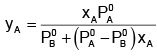Subtracting xA from both the sides, we get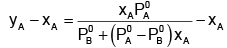Now differentiating w.r.t. xA, we get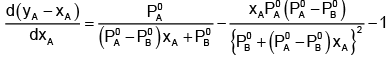The value of xA at which yA − xA has a minimum value can be obtained by putting the above derivative equal to zero. Thus we have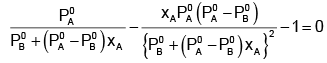Solving for xA, we get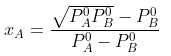hence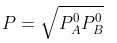Example 3. A very small amount of non-volatile solute (that does not dissociate) is dissolved in 56.8 cm3 of benzene (density 0.889 g cm-3). At room temperature, the vapour pressure of this solution is 99.88 mm Hg while that of benzene is 100mm Hg. Find the molality of this solution. If the freezing temperature of this solution is 0.73 degree lower than that of benzene. What is the value of molal freezing point depression constant of benzene?
Solution.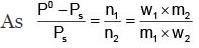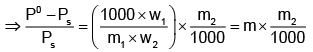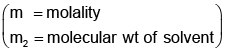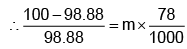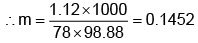Also,
ΔTf = Kf × molality f
∴ 0.73 = K × 0.1452
fK = 5.028 K. molality

Example 4. What is the composition of the vapour which is in equilibrium at 30°C with a benzene-toluene solution with a mole fraction of benzene of 0.400?
(PoB = 119 torr and PTo = 37.0 torr)
Solution.
Total pressure of the solution is given by
PT = XB P0B + XTP0T
= 0.4 × 119 + 0.6 × 37
= 47.6 + 22.2
= 69.8 torr
Applying Dalton’s law for mole fraction in vapour phase.
YB = PB/PT = 47.6/62.4
= 0.763
YT = 1– 0.763
= 0.237

TRY YOURSELF!
Q.1. If PA is the vapour pressure of a pure liquid A and the mole fraction of A in the mixture of two liquids A and B is x, then, what is the partial vapour pressure of A?
Ans. XPa

Q.2. Vapour pressure of C6H6 and C7H8 mixture at 50°C is given by P (mm Hg) = 180 XB + 90, where XB is the mole fraction of C6H6. A solution is prepared by mixing 936 g benzene and 736 g toluene and if the vapours over this solution are removed and condensed into liquid and again brought to the temperature of 50°C, what would be mole fraction of C6H6 in the vapour state?
Ans. 0.93

Q.3.When a solute is added to a pure solvent, which statement is not true?
(a) Vapour pressure of the solution becomes lower than the vapour pressure of the pure solvent.
(b) Rate of evaporation of the pure solvent is reduced.
(c) Solute does not affect the rate of condensation.
(d) None of these.
Ans. (d)

Q.4. According to Raoult's law the relative decrease in solvent vapour pressure over the solution is equal to
(a) The mole fraction of the solvent.
(b) The number of moles of the solute.
(c) 'i' times the mole fraction of the solute which undergoes dissociation or association in the solvent (i = Vant Hoff factor).
(d) None.
Ans. (c)

Offer running on EduRev: Apply code STAYHOME200 to get INR 200 off on our premium plan EduRev Infinity!

,

,

,

,

,

,

,

,

,

,

,

,

,

,

,

,

,

,

,

,

,

;